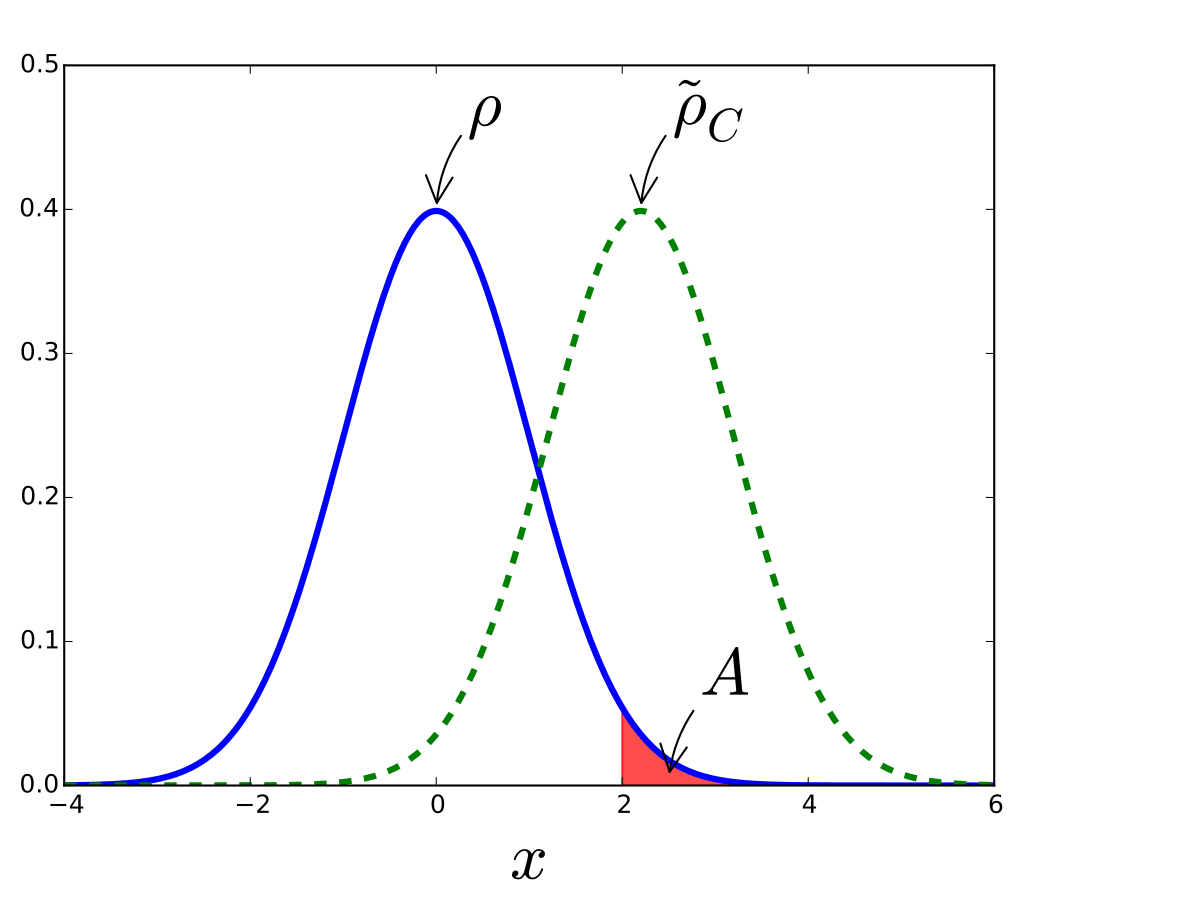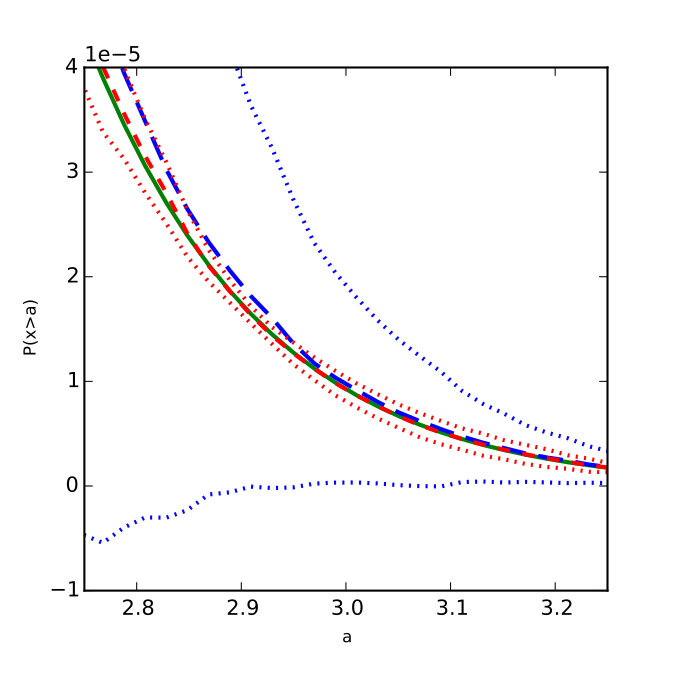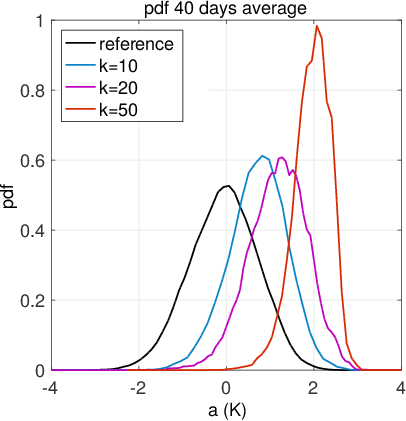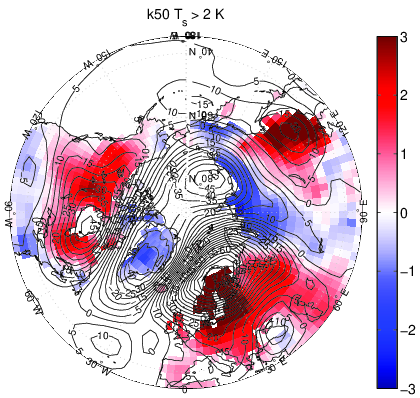Rare events are of importance in many branches of science, for example in the form of heat waves or storms in climate dynamics and meteorology or complex reactions in chemistry. Although these events are rare, they can have a large impact (see also the research project for a more in depth discussion on this issue).

In this area I have worked on the numerical simulation of rare events (Rubino & Tuffin, 2009; Del Moral, 2004) and on extreme value theory (Leadbetter, 1983; de Haan, 2006; Embrechts et al., 1997). I have developed a method to significantly improve the efficiency of numerical rare event sampling (Wouters & Bouchet, 2016). In extreme value theory I have developed new connections between geometrical properties of strange attractors and extreme value statistics (Lucarini et al., 2012). I will give a more detailed description of these two research fields and of my contributions below.

### Rare event simulation

The cost of numerically calculating probabilities of rare events rapidly becomes prohibitive as the event of interest becomes rare. Acceptable statistics for a once-in-1000-year heat wave for example require general circulation model runs of $10^5$ years, which are currently not feasible. In rare event sampling ensembles of independent realisations of a model are manipulated such that rare events become typical. In this way the algorithms use the available computational resources more efficiently, making estimation of rare events possible.

Say one wants to estimate a small probability $\gamma_A = P (X \in A) \ll 1$ by means of a brute force Monte Carlo estimate $\hat{\gamma}_A = \frac{1}{N} \sum 1_A (X_i)$. The estimator $\hat{\gamma}_A$ is an unbiased estimator of $\gamma_A$ since the expectation value of $\hat{\gamma}_A$ is clearly $\gamma_A$. The statistical error of the estimator can be quantified by its variance $\text{var} (\hat{\gamma}_A) = \text{var} (1_A (X)) / N$. Since $\text{var} (1_A (X)) = \gamma_A - \gamma_A^2 \approx \gamma_A$ when $\gamma_A$ is small, the relative error of the estimator will be $\text{RE} \sim \text{std} (\hat{\gamma}_A) / \gamma_A = 1 / \sqrt{\gamma_A N}$. It becomes apparent that the relative error quickly becomes large as $\gamma_A$ goes to zero for any finite sample size $N$.

Rare event computation techniques abate these difficulties by sampling values $Y_i$ from a modified probability measure $\tilde{\rho}$ together with an adapted estimator $\tilde{\gamma}$ to counteract this change of measure. In this case we can estimate $\gamma_A$ as $\tilde{\gamma} = \frac{1}{N} \sum_{i = 1}^N (\rho (Y_i) / \tilde{\rho} (Y_i)) (\operatorname{1}_A (Y_i))$. A short calculation shows that this is again an unbiased estimator since $\tilde{\mathbb{E}} (\tilde{\gamma} ) = \gamma_A$, where $\tilde{\mathbb{E}}$ is the expectation value with respect to $\tilde{\rho}$. Such an estimator can have a greatly reduced error. Figure 1 illustrates how the standard deviation (and therefore also the error) of the estimator $\hat{\gamma}$ decreases when the original measure $\rho$ is a normal distribution $N_{0, 1}$, the rare event set is $A = [2, \infty]$ and the altered distribution $\widetilde{\rho}$ is also normal with a shifted mean $C$, i.e. $N_{C, 1}$. The brute force estimator is equivalent to the $C = 0$ point on this plot, whereas an estimator with $C \sim 2.2$ have a much lower standard deviation (and error).Figure 1: Left plot: the orginal normal distribution $\rho = N_{0, 1}$, the shifted normal distribution $\tilde{\rho} =N_{C, 1}$ used for the rare event estimator $\tilde{\gamma}$ of the probability of the rare event set $A = [2, \infty]$ under $\rho$. Right plot: the ratio of the standard deviation of the rare event estimator $\text{std} (\tilde{\gamma})$ to the estimated probability $\gamma_A$ (quantifying the estimator error).

Note how the error decreases as the mean of the distribution $\tilde{\rho}$ is shifted and the rare event becomes typical. For illustrational purposes a set $A$ was selected that is not too rare; the improvement obtained by rare event estimation increases as the set becomes more rare.

For a dynamical process $x (t)$, instead of a random variable $X_i$, such a change of measure $\rho \rightarrow \tilde{\rho}$ can be performed in two different ways. Either the dynamics $\dot{x} = f (x)$ can be altered to include a control field $\dot{\tilde{x}} = f (\tilde{x}) + u (\tilde{x})$, thereby altering the distribution of paths of the system. The path distribution can also be changed by regularly performing selection steps, i.e. by cloning and killing realisations along a simulation of an ensemble, depending on an a weight for each member, as determined by an objective function. The former approach is called importance sampling, whereas the latter approach is called genealogical particle analysis (GPA), the “particles” being the different simulated realisations.

The objective function that determines the weight assigned to each particle in GPA is difficult to construct as it requires prior knowledge of the rare event dynamics. Ideally, particles that are likely to end up in the rare event set should be cloned and the ones that are likely to end up elsewhere should be killed. This information on where the particle ends up is however not available until the entire simulation has ended and not when it is needed, at the intermediate selection times!

My research has focused on improving the efficiency of the class of genealogical particle analysis algorithms by making use of the limiting distribution of paths in weak noise diffusions $d x = f (x) d t + \varepsilon d W$ as $\varepsilon \rightarrow 0$. For this type of system, paths to a rare event concentrate around a most likely fluctuation path, which can be calculated as a minimiser of an action functional on the paths. I have made use of this characteristic of the distribution of paths to construct a suitable objective function. The objective function is constructed such that it selects particles moving along the most likely path, which are more likely to end up in the rare event set. Figure 2 shows the drastic estimator error reduction that is possible by using this objective function instead of the simple objective function $\exp (C \Delta x)$ that has been used elsewhere, with $\Delta x$ the displacement between selections (Garnier & Del Moral, 2006).Figure 2: The estimation of a rare probability $P (x (T) > a)$ for the Ornstein-Uhlenbeck process $d x = - x d t + \text{dW}$ for a range of thresholds $a$, at $T = 2$, through GPA with the $\exp (C \Delta x)$ objective function (blue), large deviation based GPA (red) and the analytical value (green). The upper and lower dotted red and blue lines depict the $\pm 2 \sigma$ intervals. The middle dashes lines show the estimated value.

For dynamical systems more general than the weak noise diffusions I have furthermore developed a computational method to iteratively improve the objective function. With these methods I was able to vastly improve the accuracy of small probability estimates, or, equivalently, to significantly reduce the numerical cost of rare event sampling, since shorter GPA runs can attain in this way the same accuracy as longer brute force or even a naively constructed GPA simulation. The method initially uses a rough estimate of the path leading to an intermediate threshold, obtained from a short brute force simulation. This path can then be used to perform a first GPA simulation, allowing to estimate the path to a new, higher threshold, after which the GPA algorithm can be performed up to this new threshold. An article on this work has been submitted to Journal of Physics A (Wouters & Bouchet, 2016).

Together with Francesco Ragone (University of Milano-Bicocca) and Freddy Bouchet (École Normale Supérieure de Lyon), I have performed the very first genealogical particale analysis of rare events in a general circulation model (the PlaSim climate model). Using a variant of the GPA algorithm due to Giardinà, Kurchan, Lecomte, Tailleur (GKLT) and others (Giardinà et al., 2006; Tailleur & Kurchan, 2007; Lecomte & Tailleur, 2007), rare events can be estimated that are far out of reach of brute force calculations (see Figure 3). These first results on European heat waves confirm the connection between blocking high pressure patterns and heat wave conditions that has been proposed in the meteorology literature and demonstrates a new teleconnection pattern during heat wave conditions. A paper describing these results has been published in PNAS (Ragone et al., 2017).Figure 3: Rare event simulation on the Planet Simulator climate model, using a genealogical particle analysis algorithm. Top: by increasing a parameter $k$, the temperature averages (here averaged over Europe and a 40 day time interval) can be controlled, making heat waves that are very rare in the reference climate common. Bottom: this allows for a very accurate estimation of for example the atmospheric flow pattern related to very rare heat waves.

### Extreme value theory

Extreme value theory is a field of statistics describing the distributions of extrema of random variables. The theory describes the asymptotic distribution of extremes of a series of random variables $X_i \; i \in { 0, \ldots, n }$. The extremes can be described either by maxima $\max_i (X_i)$ (the so-called block maxima approach) or by conditional over-threshold probabilities $P (X_0 > a + y|X_0 > a)$ (the peak-over-threshold approach). As the length of the block $n$ or the threshold $a$ is taken to infinity, the two distributions converge, to the generalized extreme value and generalized Pareto distributions respectively (Leadbetter, 1983). The two approaches are closely related in the sense that convergence of the block maxima distribution as $n \rightarrow \infty$ holds if and only if the over-threshold distributions hold as $a \rightarrow \infty$ and the parameters of the two limit distributions are directly related (Lucarini et al., 2016). The great interest in this theory in physics and climatology derives from the fact that it can be used to fit distributions of observed maxima (for example the maxima of rainfall data (Deidda, 2010)), thereby predicting the probability of as of yet unobserved extremes (Embrechts et al., 1997). The theory is well developed for independent and weakly dependent random variables; application of this theory to deterministic dynamical systems is however relatively new.

With Valerio Lucarini, Tobias Kuna (University of Reading) and Davide Faranda (University of Paris-Saclay) I have developed new connections between extreme value theory and the dimension theory for strange attractors. By using the peak-over-threshold approach, we have derived relations between the parameters of the extreme value laws and dynamical properties of the underlying dynamical system, in particular the attractor dimension of the attractor (Lucarini et al., 2012; Lucarini et al., 2014). These relations depend on the type of observable and the partial dimensions of the attractor on the stable and unstable manifolds. This connection can therefore be used to improve estimation of extreme value distributions if estimates of the attractor dimensions are available from standard algorithms such as the Grassberger-Procaccia algorithm. Our work also explains the observed smooth dependence of extreme value parameters on the parameters of the dynamical system in intermediate complexity atmospheric models (see references in (Lucarini et al., 2014)), since attractor dimensions are observed to be insensitive to parameter changes (Albers & Sprott, 2006).

Together with Paul Manneville (École Polytechnique, Paris) we have derived a method based on extreme value theory to detect dynamical transitions in turbulent flows, such as the Couette flow (Faranda et al., 2014). Our method removes the ambiguity of selecting an arbitrary threshold as required in the standard autocorrelation or moment based methods (Kuehn, 2011). We have exploited the fact that upon transitioning from sustained to transient turbulence the system makes large excursions to low-energy states, giving the energy distribution a fatter lower tail. Such fat-tailed underlying probability distributions result in distributions of block minima according to a so called Fréchet distribution, featuring a positive shape parameter. In the sustained turbulence state on the other hand the energy distribution is lower bounded and therefore the minima are Weibull distributed, with a negative shape parameter. The transition can then be readily detected as a simple change in sign of the extreme value distribution shape parameter. Our method has the distinct advantage of having a clear threshold for the parameter where a transition is likely to occur, namely zero. This contrasts to methods based on an increase in variance or skewness that do not have such a threshold. This advantage of the extremes based approach makes it more readily applicable and it has since been succesfully applied to estimate transition thresholds in an experiment of a dynamo magnetic field (Faranda et al., 2014).

1. Rubino, G., & Tuffin, B. (Eds.). (2009). Rare event simulation using Monte Carlo methods. Wiley.
2. Del Moral, P. (2004). Feynman-Kac Formulae Genealogical and Interacting Particle Systems with Applications. Springer New York.
3. Leadbetter, M. R. (1983). Extremes and related properties of random sequences and processes. Springer-Verlag.
4. de Haan, L. (2006). Extreme value theory: an introduction. Springer.
5. Embrechts, P., Klüppelberg, C., & Mikosch, T. (1997). Modelling Extremal Events for Insurance and Finance. Springer Berlin Heidelberg.
6. Wouters, J., & Bouchet, F. (2016). Rare Event Computation in Deterministic Chaotic Systems Using Genealogical Particle Analysis. Journal of Physics A: Mathematical and Theoretical, 49(37), 374002. https://doi.org/10.1088/1751-8113/49/37/374002
7. Lucarini, V., Faranda, D., & Wouters, J. (2012). Universal Behaviour of Extreme Value Statistics for Selected Observables of Dynamical Systems. Journal of Statistical Physics, 147(1), 63–73. https://doi.org/10.1007/s10955-012-0468-z
8. Garnier, J., & Del Moral, P. (2006). Simulations of rare events in fiber optics by interacting particle systems. Optics Communications, 267(1), 205–214. https://doi.org/10.1016/j.optcom.2006.05.066
9. Giardinà, C., Kurchan, J., & Peliti, L. (2006). Direct Evaluation of Large-Deviation Functions. Physical Review Letters, 96(12), 120603. https://doi.org/10.1103/PhysRevLett.96.120603
10. Tailleur, J., & Kurchan, J. (2007). Probing rare physical trajectories with Lyapunov weighted dynamics. Nature Physics, 3(3), 203–207. https://doi.org/10.1038/nphys515
11. Lecomte, V., & Tailleur, J. (2007). A numerical approach to large deviations in continuous time. Journal of Statistical Mechanics: Theory and Experiment, 2007(03), P03004. https://doi.org/10.1088/1742-5468/2007/03/P03004
12. Ragone, F., Wouters, J., & Bouchet, F. (2017). Computation of extreme heat waves in climate models using a large deviation algorithm. Proceedings of the National Academy of Sciences, 201712645. https://doi.org/10.1073/pnas.1712645115
13. Lucarini, V., Faranda, D., de Freitas, A. C. G. M. M., de Freitas, J. M. M., Vaienti, S., Todd, M., Nicol, M., Kuna, T., & Holland, M. P. (2016). Extremes and Recurrence in Dynamical Systems (1 edition). Wiley.
14. Deidda, R. (2010). A multiple threshold method for fitting the generalized Pareto distribution to rainfall time series. Hydrol. Earth Syst. Sci., 14(12), 2559–2575. https://doi.org/10.5194/hess-14-2559-2010
15. Lucarini, V., Faranda, D., Wouters, J., & Kuna, T. (2014). Towards a General Theory of Extremes for Observables of Chaotic Dynamical Systems. Journal of Statistical Physics, 154(3), 723–750. https://doi.org/10.1007/s10955-013-0914-6
16. Albers, D. J., & Sprott, J. C. (2006). Structural stability and hyperbolicity violation in high-dimensional dynamical systems. Nonlinearity, 19(8), 1801–1847. https://doi.org/10.1088/0951-7715/19/8/005
17. Faranda, D., Lucarini, V., Manneville, P., & Wouters, J. (2014). On using extreme values to detect global stability thresholds in multi-stable systems: The case of transitional plane Couette flow. Chaos, Solitons & Fractals, 64, 26–35. https://doi.org/10.1016/j.chaos.2014.01.008
18. Kuehn, C. (2011). A mathematical framework for critical transitions: Bifurcations, fast–slow systems and stochastic dynamics. Physica D: Nonlinear Phenomena, 240(12), 1020–1035. https://doi.org/10.1016/j.physd.2011.02.012
19. Faranda, D., Bourgoin, M., Miralles, S., Odier, P., Pinton, J.-F., Nicolas Plihon, Daviaud, F., & Dubrulle, B. (2014). Robust estimate of dynamo thresholds in the von Kármán sodium experiment using the extreme value theory. New Journal of Physics, 16(8), 083001. https://doi.org/10.1088/1367-2630/16/8/083001